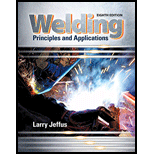Chapter 11, Problem 13R### Welding: Principles and Applicatio...

8th Edition
Larry Jeffus
ISBN: 9781305494695

#### Solutions

Chapter
Section### Welding: Principles and Applicatio...

8th Edition
Larry Jeffus
ISBN: 9781305494695
Textbook Problem
45 views

# Using Table 11-1, determine the amperage if 400 ipm (10.2 m/min) of 0.45-in (1.2-mm) steel wire is fed in 1 minute.

To determine

The value of amperage.

Explanation

Given:

The diameter of electrode is 0.045in.

The speed of wire is 400ipm.

Concept used:

The Amperage of electrode can be defined as the strength of electric current required to fuse the metal by melting the electrode and base metal.

To calculate the amperage from table 11.1, follow the below mentioned steps:

1. Make the corresponding value of diameter of electrode for which the amperage needs to be determined.
2. Make the type of arc required for GMAW welding. As in this case it is spray metal arc.
3. There are three values for the amperage.
4. The middle value of the amperage is the typical value for particular diameter and it can be considered as general value of amperage

### Still sussing out bartleby?

Check out a sample textbook solution.

See a sample solution

#### The Solution to Your Study Problems

Bartleby provides explanations to thousands of textbook problems written by our experts, many with advanced degrees!

Get Started

#### Why must a band saw blade be annealed after welding?

Precision Machining Technology (MindTap Course List)

#### What are the two most common systems of units?

Engineering Fundamentals: An Introduction to Engineering (MindTap Course List)

#### Write the business rules reflected in this ERD.

Database Systems: Design, Implementation, & Management